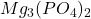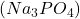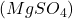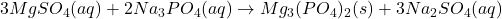## When an aqueous solution of sodium phosphate (Na3PO4) and an aqueous solution of magnesium sulfate (MgSO4) are mixed, a precipitate forms. W

Question

When an aqueous solution of sodium phosphate (Na3PO4) and an aqueous solution of magnesium sulfate (MgSO4) are mixed, a precipitate forms. Write the chemical formula of the precipitate.

in progress 0
6 months 2021-07-28T02:50:19+00:00 1 Answers 291 views 0

1. Answer: The chemical formula of this precipitate is.

Explanation:

An equation which depicts the chemical reaction of substances in the form of chemical formulas is called a chemical equation.

For example, chemical equation for an aqueous solution of sodium phosphateand an aqueous solution of magnesium sulfateare mixed, a precipitate forms is as follows.Here, trimagnesium phosphate is the precipitate. The chemical formula of this precipitate is.

Thus, we can conclude that the chemical formula of this precipitate is.